# Stoichiometry The calculation of quantities in chemical equations

• Slides: 26Stoichiometry • The calculation of quantities in chemical equations. • Interpreting balanced chemical equations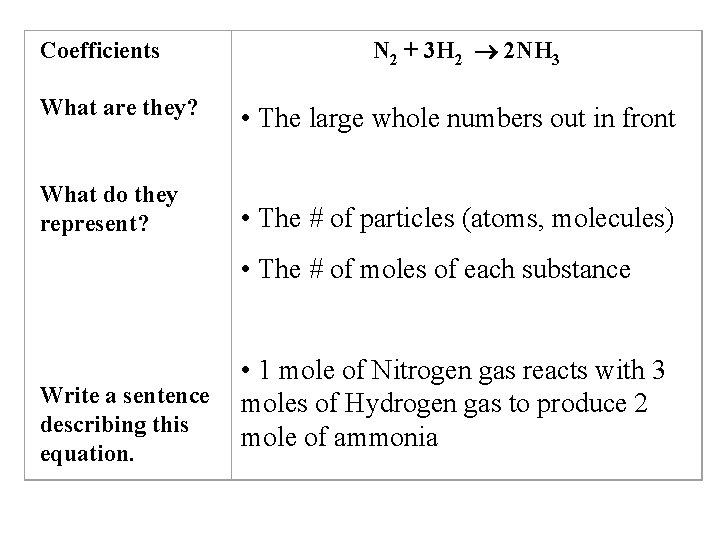Coefficients N 2 + 3 H 2 2 NH 3 What are they? • The large whole numbers out in front What do they represent? • The # of particles (atoms, molecules) • The # of moles of each substance Write a sentence describing this equation. • 1 mole of Nitrogen gas reacts with 3 moles of Hydrogen gas to produce 2 mole of ammonia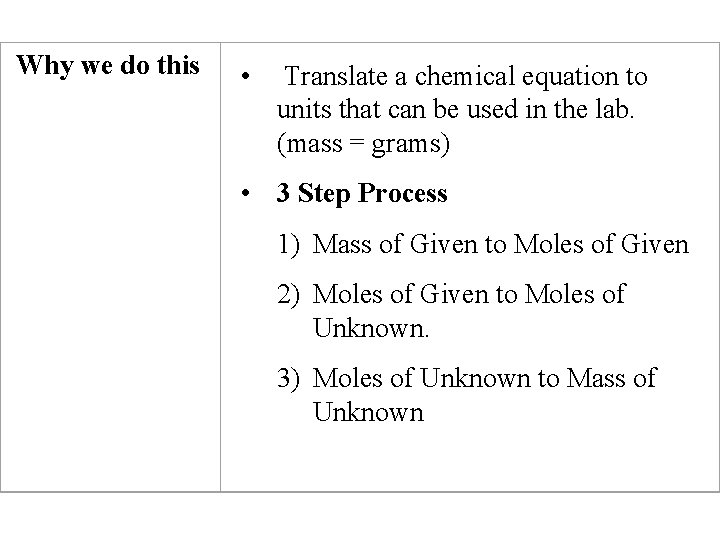Why we do this • Translate a chemical equation to units that can be used in the lab. (mass = grams) • 3 Step Process 1) Mass of Given to Moles of Given 2) Moles of Given to Moles of Unknown. 3) Moles of Unknown to Mass of UnknownMole Calculations • Converting from moles of one substance to moles of another, using a balanced chemical equation.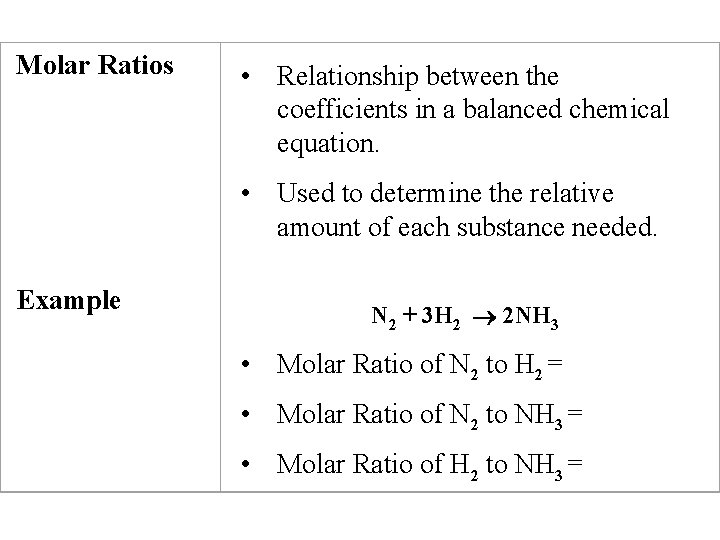Molar Ratios • Relationship between the coefficients in a balanced chemical equation. • Used to determine the relative amount of each substance needed. Example N 2 + 3 H 2 2 NH 3 • Molar Ratio of N 2 to H 2 = • Molar Ratio of N 2 to NH 3 = • Molar Ratio of H 2 to NH 3 =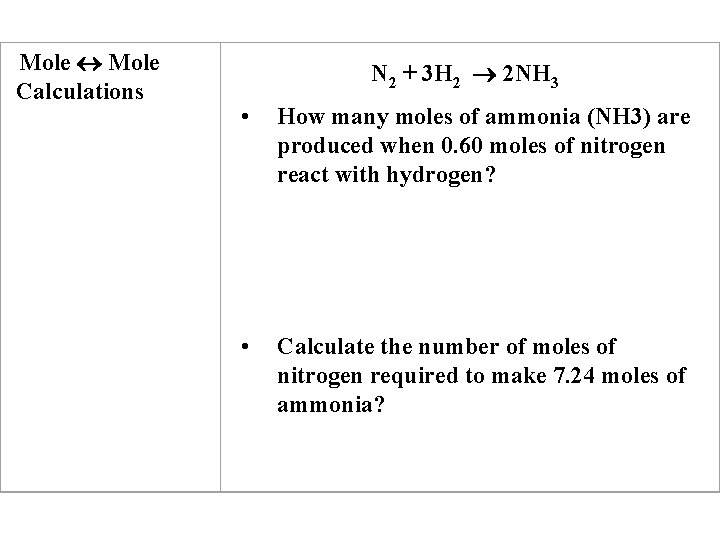Mole Calculations N 2 + 3 H 2 2 NH 3 • How many moles of ammonia (NH 3) are produced when 0. 60 moles of nitrogen react with hydrogen? • Calculate the number of moles of nitrogen required to make 7. 24 moles of ammonia?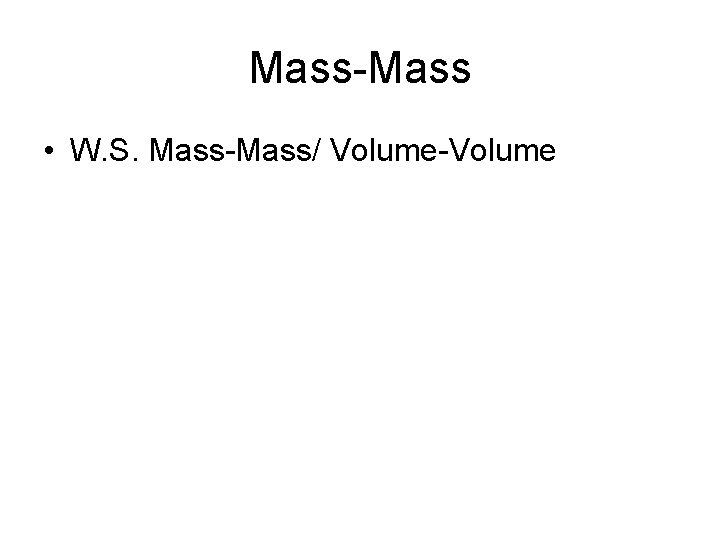Mass-Mass • W. S. Mass-Mass/ Volume-VolumeModel 3: The Balanced Chemical EquationBrain Teaser • Be + 2 HCl Be. Cl 2 + H 2 • Given 1. 20 g of Be, how many grams of Beryllium Chloride was produced? If my actual yield was 4. 5 grams, what was my percent yield?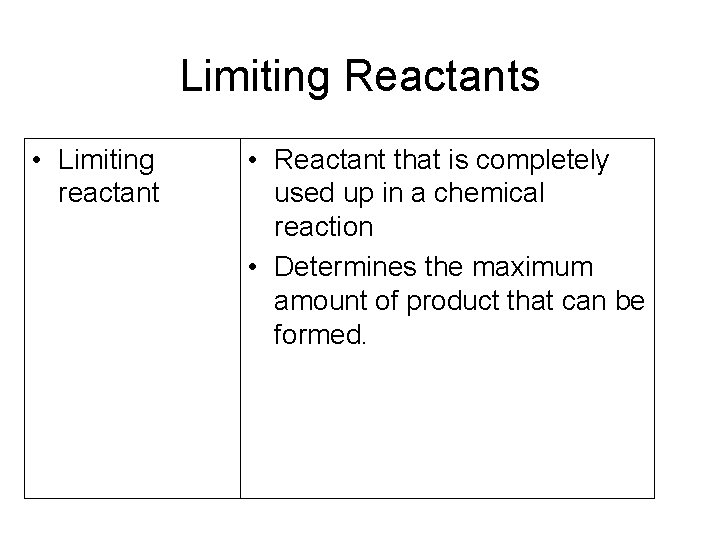Limiting Reactants • Limiting reactant • Reactant that is completely used up in a chemical reaction • Determines the maximum amount of product that can be formed.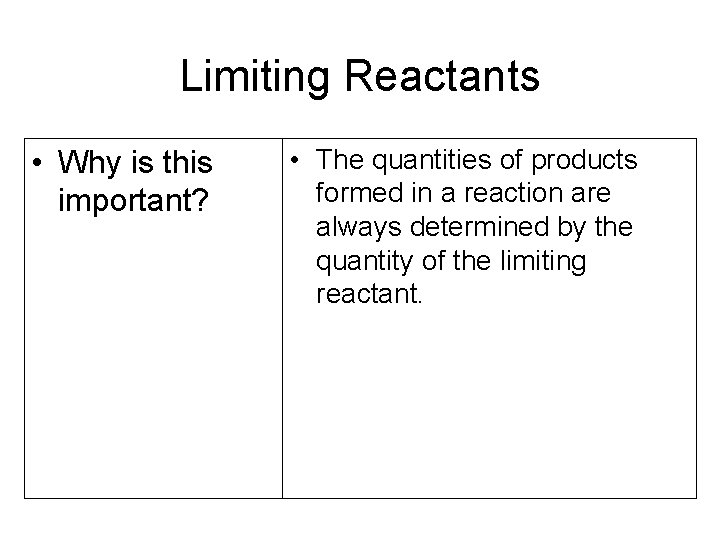Limiting Reactants • Why is this important? • The quantities of products formed in a reaction are always determined by the quantity of the limiting reactant.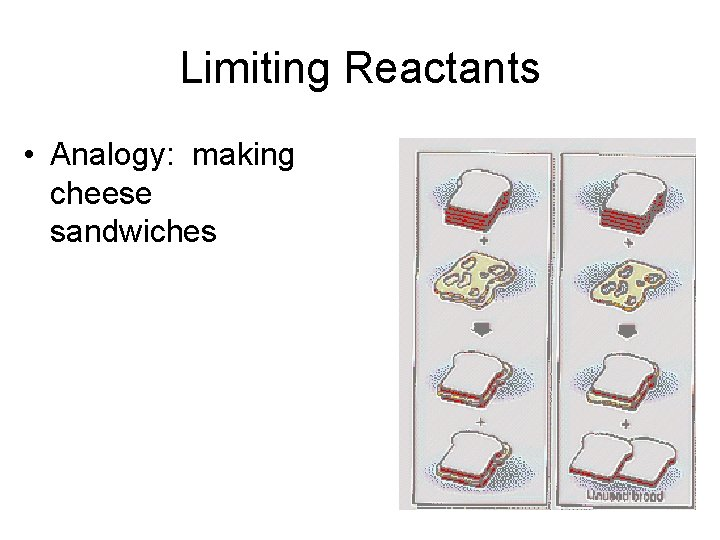Limiting Reactants • Analogy: making cheese sandwiches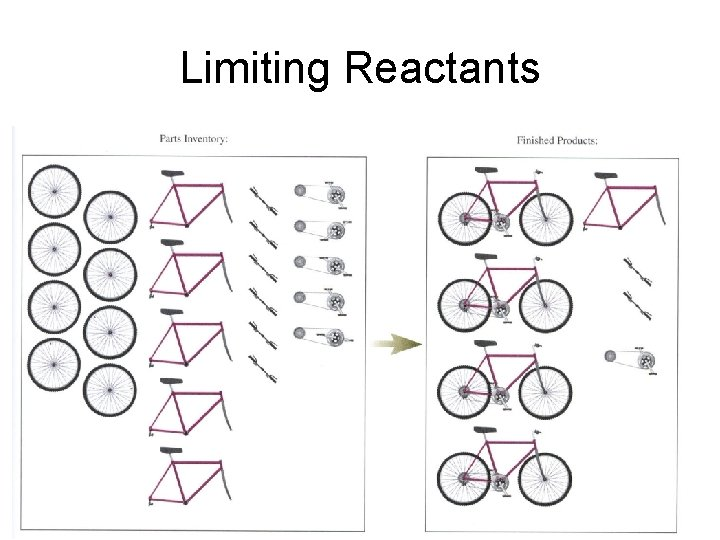Limiting Reactants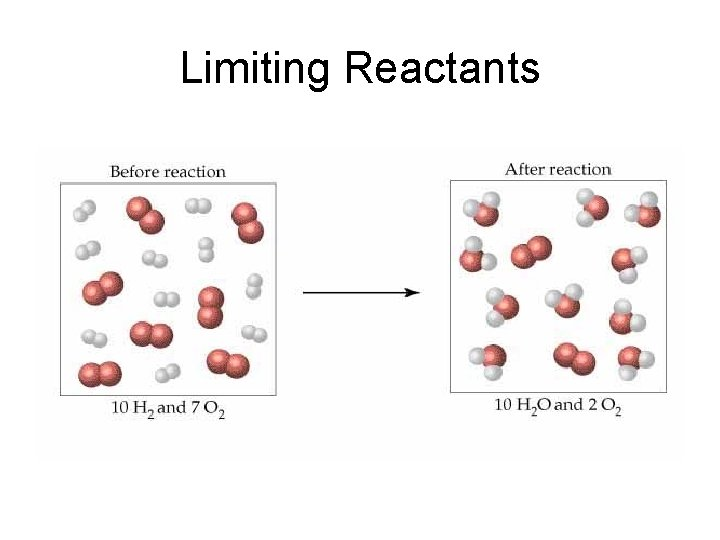Limiting ReactantsLimiting Reactants 2 H 2 + O 2 2 H 2 O What is the limiting reactant?Limiting Reactants 2 H 2 + O 2 2 H 2 O What is the limiting reactant?Limiting Reactants 2 H 2 + O 2 2 H 2 O What is the limiting reactant?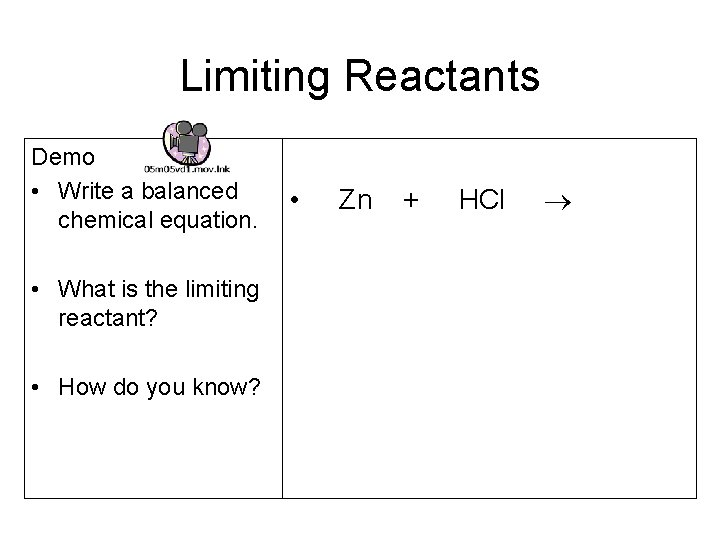Limiting Reactants Demo • Write a balanced chemical equation. • What is the limiting reactant? • How do you know? • Zn + HCl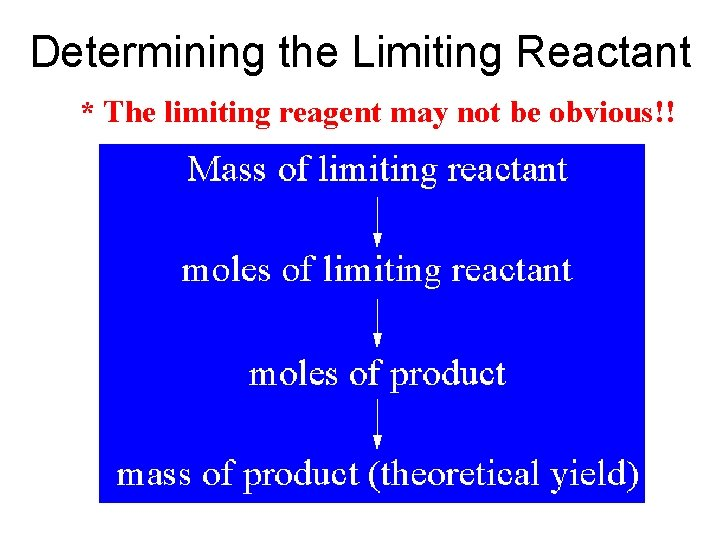Determining the Limiting Reactant * The limiting reagent may not be obvious!!Example • If 3. 5 grams of copper are added to a solution containing 6. 0 grams of silver nitrate. What is the limiting reagent and how much silver can be produced? Cu + 2 Ag. NO 3 Cu(NO 3)2 + 2 Ag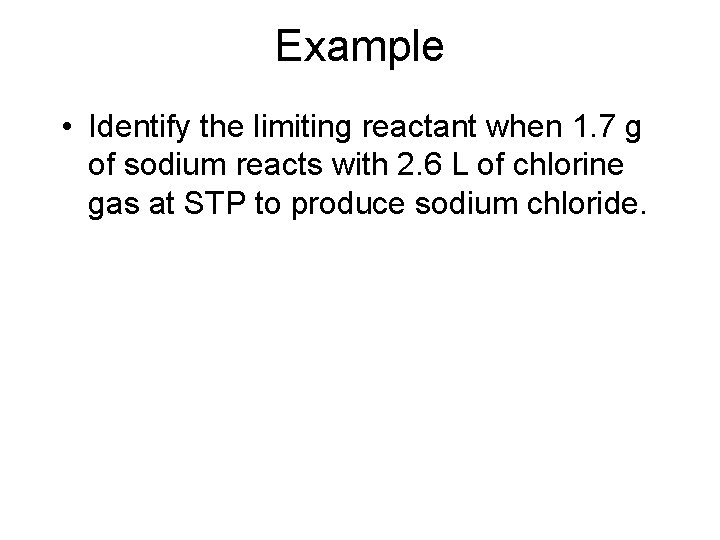Example • Identify the limiting reactant when 1. 7 g of sodium reacts with 2. 6 L of chlorine gas at STP to produce sodium chloride.Quiz 1) Ca. Cl 2 + Na 2 CO 3 Ca. CO 3 + 2 Na. Cl 5. 45 g 4. 55 g ? ? 111 g/mol 106 g/mol 100. 1 g/mol 58. 4 g/mol How much Ca. CO 3 (in g) is formed? 2) Ca. Cl 2 + Na 2 CO 3 Ca. CO 3 + 2 Na. Cl 0. 105 mol 0. 085 mol ? ? How many moles of each product could be produced?Can you really get out everything that goes in? % yield = experimental yield x 100 theoretical yield Experimental or actual yield determined in the lab Theoretical or expected yield determined by stoichiometryGroup Quiz 1) Ca. Cl 2 + Na 2 CO 3 Ca. CO 3 + 2 Na. Cl 0. 105 mol 36. 8 L at STP ? ? How many moles of each product could be produced? 2) Ca. Cl 2 + Na 2 CO 3 Ca. CO 3 + 2 Na. Cl 5. 45 g 4. 55 g ? How much Ca. CO 3 (in g) is formed? What is the percent yield for this reaction if 3. 96 g of calcium carbonate was isolated from the reaction?How efficient are you in the lab? • Work as a team • Each group member must perform one of the reactions. • Calculate each individuals efficiency as well as your group average. • Each group member is responsible for their own lab report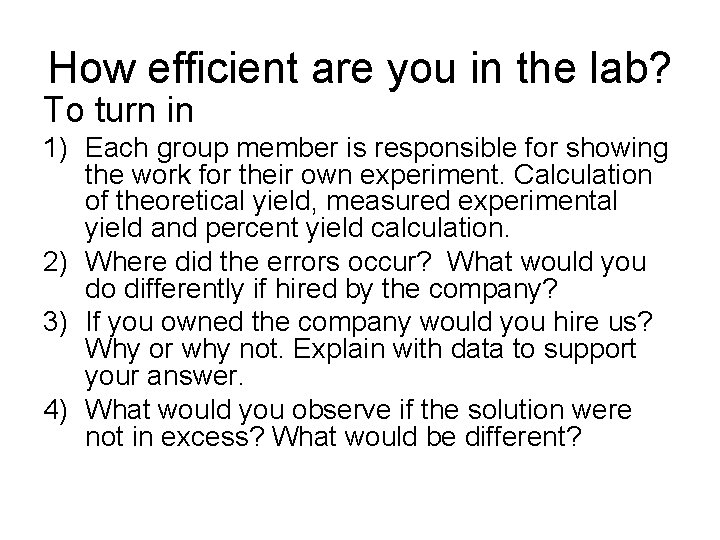How efficient are you in the lab? To turn in 1) Each group member is responsible for showing the work for their own experiment. Calculation of theoretical yield, measured experimental yield and percent yield calculation. 2) Where did the errors occur? What would you do differently if hired by the company? 3) If you owned the company would you hire us? Why or why not. Explain with data to support your answer. 4) What would you observe if the solution were not in excess? What would be different?